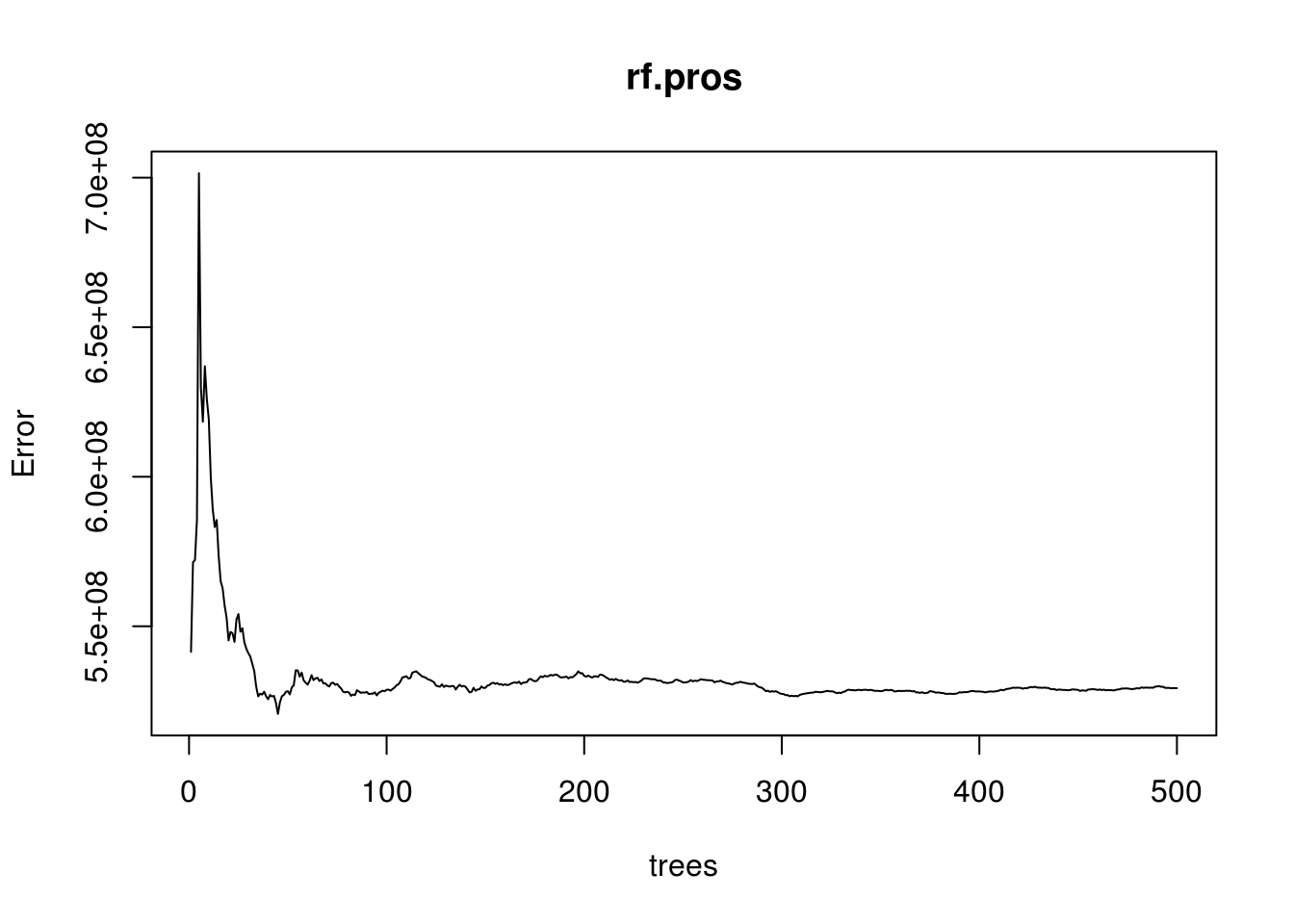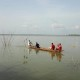# Random Forest Regression Trees in R

Random forest involves the process of creating multiple decision trees and the combing of their results. How this is done is through r using 2/3 of the data set to develop decision tree. This is done dozens, hundreds, or more times. Every tree made is created with a slightly different sample. The results of all these trees are then averaged together. This process of sampling is called bootstrap aggregation or bagging for short.While the random forest algorithm is developing different samples it also randomly selects which variables to be used in each tree that is developed. By randomizing the sample and the features used in the tree, random forest is able to reduce both bias and variance in a model. In addition, random forest is robust against outliers and collinearity. Lastly, keep in mind that random forest can be used for regression and classification trees

In our example, we will use the “Participation” dataset from the “Ecdat” package. We will create a random forest regression tree to predict income of people. Below is some initial code

``library(randomForest);library(rpart);library(Ecdat)``
``````data("Participation")
str(Participation)``````
``````## 'data.frame':    872 obs. of  7 variables:
##  \$ lfp    : Factor w/ 2 levels "no","yes": 1 2 1 1 1 2 1 2 1 1 ...
##  \$ lnnlinc: num  10.8 10.5 11 11.1 11.1 ...
##  \$ age    : num  3 4.5 4.6 3.1 4.4 4.2 5.1 3.2 3.9 4.3 ...
##  \$ educ   : num  8 8 9 11 12 12 8 8 12 11 ...
##  \$ nyc    : num  1 0 0 2 0 0 0 0 0 0 ...
##  \$ noc    : num  1 1 0 0 2 1 0 2 0 2 ...
##  \$ foreign: Factor w/ 2 levels "no","yes": 1 1 1 1 1 1 1 1 1 1 ...``````

We now need to prepare the data. We need to transform the lnnlinc from a log of salary to the actual salary. In addition, we need to multiply “age” by ten as 3.4 & 4.5 do not make any sense. Below is the code

``````Participation\$age<-10*Participation\$age #normal age
Participation\$lnnlinc<-exp(Participation\$lnnlinc) #actual income not log``````

Now we create our training and testing sets.

``````set.seed(123)
ind=sample(2,nrow(Participation),replace=T,prob=c(.7,.3))
train<-Participation[ind==1,]
test<-Participation[ind==2,]``````

We are now ready to create our model. Below is the code

``````set.seed(123)
rf.pros<-randomForest(lnnlinc~.,data = train)
rf.pros``````
``````##
## Call:
##  randomForest(formula = lnnlinc ~ ., data = train)
##                Type of random forest: regression
##                      Number of trees: 500
## No. of variables tried at each split: 2
##
##           Mean of squared residuals: 529284177
##                     % Var explained: 13.74``````

As you can see from calling “rf.pros” the variance explained is low at around 14%. The output also tells us how many trees were created. You have to be careful with making too many trees as this leads to overfitting. We can determine how many trees are optimal by looking at a plot and then using the “which.min”. Below is a plot of the number of trees by the mean squared error.

``plot(rf.pros)``As you can see, as there are more trees there us less error to a certain point. It looks as though about 50 trees is enough. To confirm this guess, we used the “which.min” function. Below is the code

``which.min(rf.pros\$mse)``
``##  45``

We need 45 trees to have the lowest error. We will now rerun the model and add an argument called “ntrees” to indicating the number of trees we want to generate.

``````set.seed(123)
rf.pros.45<-randomForest(lnnlinc~.,data = train,ntree=45)
rf.pros.45``````
``````##
## Call:
##  randomForest(formula = lnnlinc ~ ., data = train, ntree = 45)
##                Type of random forest: regression
##                      Number of trees: 45
## No. of variables tried at each split: 2
##
##           Mean of squared residuals: 520705601
##                     % Var explained: 15.13``````

This model is still not great. We explain a little bit more of the variance and the error decreased slightly. We can now see which of the features in our model are the most useful by using the “varImpPlot” function. Below is the code.

``varImpPlot(rf.pros.45)``The higher the IncNodePurity the more important the variable. AS you can see, education is most important followed by age and then the number of older children. The raw scores for each variable can be examined using the “importance” function. Below is the code.

``importance(rf.pros.45)``
``````##         IncNodePurity
## lfp       16678498398
## age       66716765357
## educ      72007615063
## nyc        9337131671
## noc       31951386811
## foreign   10205305287``````

We are now ready to test our model with the test set. We will then calculate the residuals and the mean squared error

``````rf.pros.test<-predict(rf.pros.45,newdata = test)
rf.resid<-rf.pros.test-test\$lnnlinc
mean(rf.resid^2)``````
``##  381850711``

Remember that the mean squared error calculated here is only useful in comparison to other models. Random forest provides a way in which to remove the weaknesses of one decision tree by averaging the results of many. This form of ensemble learning is one of the more powerful algorithms in machine learning.

## 2 thoughts on “Random Forest Regression Trees in R”

1.Adam

Thanks! very clear and informative!

2.Rashid Yakubu

Hello,
Why did you not try changing the mtry value to increase the number of variables tried for each tree?